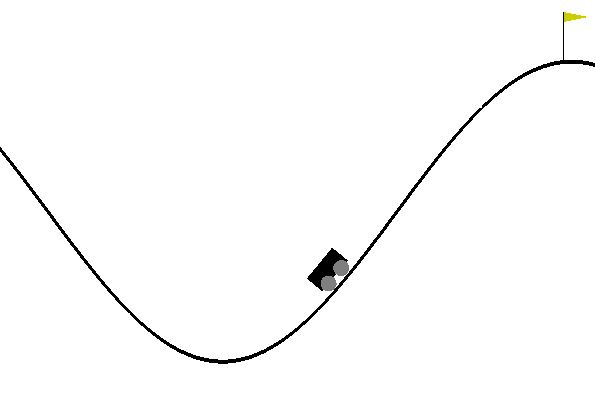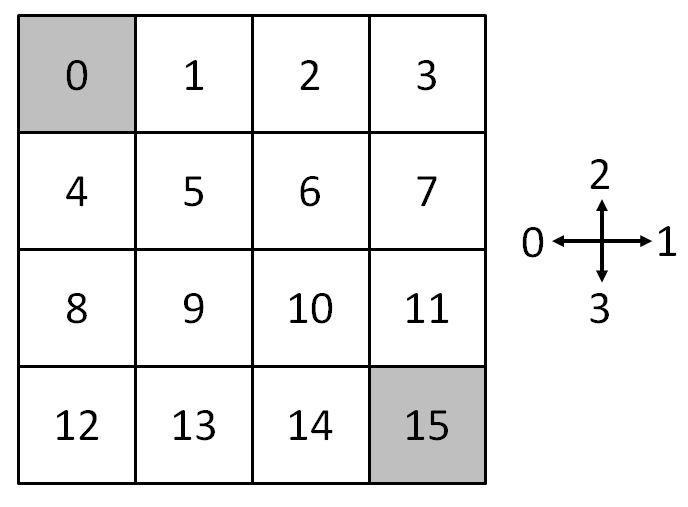This vignette explains the different possibilities to create and use a reinforcement learning environment in reinforcelearn. Section Creation explains how to create an environment and Section Interaction describe how to use the created environment object for interaction.

library(reinforcelearn)

Creation

The makeEnvironment function provides different ways to create an environment. It is called with the class name as a first argument. You can pass arguments of the specific environment class (e.g. the state transition array for an MDP) to the ... argument.

Create a custom environment

To create a custom environment you have to set up a step and reset function, which define the rewards the agent receives and ultimately the goal of what to learn.

Here is an example setting up a the famous Mountain Car problem.The task of the reset function is to initialize the starting state of the environment and usually this function is called when starting a new episode. It returns the state of the environment. It takes an argument self, which is the newly created R6 class and can be used e.g. to access the current state of the environment.

reset = function(self) {
position = runif(1, -0.6, -0.4)
velocity = 0
state = matrix(c(position, velocity), ncol = 2)
state
}

The step function is used for interaction, it controls the transition to the next state and reward given an action. It takes self and action as an argument and returns a list with the next state, reward and whether an episode is finished (done).

step = function(self, action) {
position = self$state velocity = self$state
velocity = (action - 1L) * 0.001 + cos(3 * position) * (-0.0025)
velocity = min(max(velocity, -0.07), 0.07)
position = position + velocity
if (position < -1.2) {
position = -1.2
velocity = 0
}
state = matrix(c(position, velocity), ncol = 2)
reward = -1
if (position >= 0.5) {
done = TRUE
reward = 0
} else {
done = FALSE
}
list(state, reward, done)
}

Then we can create the environment with

env = makeEnvironment(step = step, reset = reset)

OpenAI Gym

OpenAI Gym (Brockman et al. 2016) provides a set of environments, which can be used for benchmarking.

To use a gym environment you have to install

Then you can create a gym environment by passing on the name of the environment.

# Create a gym environment.
env = makeEnvironment("gym", gym.name = "MountainCar-v0")

Have a look at https://gym.openai.com/envs for possible environments.

Markov Decision Process

A Markov Decision Process (MDP) is a stochastic process, which is commonly used for reinforcement learning environments. When the problem can be formulated as a MDP, all you need to pass to makeEnvironment is the state transition array $$P^a_{ss'}$$ and reward matrix $$R_s^a$$ of the MDP.

We can create a simple MDP with 2 states and 2 actions with the following code.

# State transition array
P = array(0, c(2, 2, 2))
P[, , 1] = matrix(c(0.5, 0.5, 0, 1), 2, 2, byrow = TRUE)
P[, , 2] = matrix(c(0.1, 0.9, 0, 1), 2, 2, byrow = TRUE)

# Reward matrix
R = matrix(c(5, 10, -1, 2), 2, 2, byrow = TRUE)

env = makeEnvironment("mdp", transitions = P, rewards = R)

Gridworld

A gridworld is a simple MDP navigation task with a discrete state and action space. The agent has to move through a grid from a start state to a goal state. Possible actions are the standard moves (left, right, up, down) or could also include the diagonal moves (leftup, leftdown, rightup, rightdown).

Here is an example of a 4x4 gridworld (Sutton and Barto 2017, Example 4.1) with two terminal states in the lower right and upper left of the grid. Rewards are - 1 for every transition until reaching a terminal state.The following code creates this gridworld.

# Gridworld Environment (Sutton & Barto (2017) Example 4.1)
env = makeEnvironment("gridworld", shape = c(4, 4), goal.states = c(0, 15))

Interaction

makeEnvironment returns an R6 class object which can be used for the interaction between agent and environment.

env = makeEnvironment("gridworld", shape = c(4, 4),
goal.states = 0L, initial.state = 15L)

To take an action you can call the step(action) method. It is called with an action as an argument and internally computes the following state, reward and whether an episode is finished (done).

# The initial state of the environment.
env$reset() #>  15 env$visualize()
#>  - - - -
#>  - - - -
#>  - - - -
#>  - - - o
#>

# Actions are encoded as integers.
env$step(0L) #>$state
#>  14
#>
#> $reward #>  -1 #> #>$done
#>  FALSE

env$visualize() #> - - - - #> - - - - #> - - - - #> - - o - #> # But can also have character names. env$step("left")
#> $state #>  13 #> #>$reward
#>  -1
#>
#> $done #>  FALSE env$visualize()
#>  - - - -
#>  - - - -
#>  - - - -
#>  - o - -
#>

Note that the R6 class object changes whenever calling step or reset! Therefore calling step with the same action twice will most likely return different states and rewards!

Note also that all discrete states and actions are numerated starting with 0 to be consistent with OpenAI Gym!

The environment object often also contains information about the number of states and actions or the bounds in case of a continuous space.

env = makeEnvironment("mountain.car")
env$n.actions #>  3 env$state.space.bounds
#> []
#>  -1.2  0.5
#>
#> []
#>  -0.07  0.07

It also contains a counter of the number of interactions, i.e. the number of times step has been called, the number of steps in the current episode, the number of episodes and return in the current episode.

env = makeEnvironment("gridworld", shape = c(4, 4),
goal.states = 0L, initial.state = 15L, discount = 0.99)

env$step("up") #>$state
#>  11
#>
#> $reward #>  -1 #> #>$done
#>  FALSE
env$n.step #>  1 env$episode.return
#>  -1

env$step("left") #>$state
#>  10
#>
#> $reward #>  -1 #> #>$done
#>  FALSE
env$n.step #>  2 env$episode.return
#>  -1.99

Full list of attributes and methods:

Here is a full list describing the attributes of the R6 class created by makeEnvironment.

Attributes:

• state [any]: The current state observation of the environment. Depending on the problem this can be anything, e.g. a scalar integer, a matrix or a list.

• reward [integer(1)]: The current reward of the environment. It is always a scalar numeric value.

• done [logical(1)]: A logical flag specifying whether an episode is finished.

• discount [numeric(1) in [0, 1]]: The discount factor.

• n.step [integer(1)]: Number of steps, i.e. number of times \$step() has been called.

• episode.step [integer(1)]: Number of steps in the current episode. In comparison to n.step it will be reset to 0 when reset is called. Each time step is called it is increased by 1.

• episode.return [numeric(1)]: The return in the current episode. Each time step is called the discounted reward is added. Will be reset to 0 when reset is called.

• previous.state [any]: The previous state of the environment. This is often the state which is updated in a reinforcement learning algorithm.

Methods:

• reset(): Resets the environment, i.e. it sets the state attribute to a starting state and sets the done flag to FALSE. It is usually called at the beginning of an episode.

• step(action): The interaction function between agent and environment. step is called with an action as an argument. It then takes the action and internally computes the following state, reward and whether an episode is finished and returns a list with state, reward and done.

• visualize(): Visualize the current state of the environment.